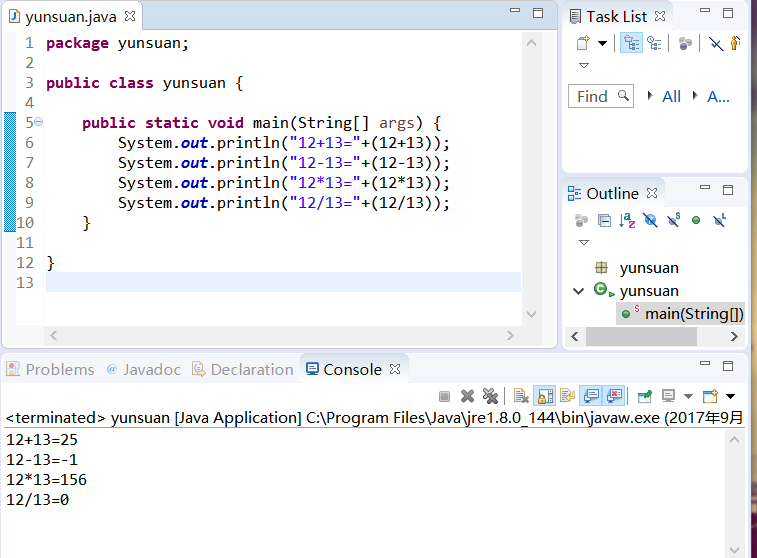• 数据结构课程设计-大整数加减乘除运算的实现，包含源代码和实验报告，解压后可直接使用
• Java简单的 整数加减乘除运算
作为一个Java小白，上传的东西都是自己的学习过程，也是最基础的。

运行结果如下图。展开全文• java计算器 可实现整数加减乘除运算，有界面。JAVA 计算器
• 文章目录只用位运算不用算术运算实现整数加减乘除运算位运算实现整型算术运算加法相应代码减法相应代码乘法相应代码除法相应代码 只用位运算不用算术运算实现整数加减乘除运算 位运算实现整型算术运算 【题目】 ...
• 用C++ 实现大整数加减乘除运算，里面有很多值得学习的技巧
• vc6.0环境，单位整数加减乘除，支持连加、连减、混合运算。不支持括号。实现方法简单，运行可靠。
• 掌握大整数的基本思想，并运用大整数的基本运算计算出常规整数n的阶乘，然后统计大整数n!中数字0的个数。 相关知识 为了完成本关任务，你需要掌握：1.大整数的思想，2.大整数加法，3.大整数减法，4.大整数整数的...
本关任务：
掌握大整数的基本思想，并运用大整数的基本运算计算出常规整数n的阶乘，然后统计大整数n!中数字0的个数。
相关知识
为了完成本关任务，你需要掌握：1.大整数的思想，2.大整数加法，3.大整数减法，4.大整数与整数的乘法，5.大整数乘法，6.大整数与整数的除法，7.n的阶乘求解思路。
大整数的思想
大整数的思想：用数组存储大整数（超长整数），为处理简单起见约定每个数组元素存放相同位数（T位）的数字片段（假定T=4位）。
设定一个大小为N的整型数组a[0,1,…,N−1]，给定一个大整数998877665544332211，每个数组单元使用T=4位存储，为了方便计算，将大整数的低位存入数组的高维索引，如下图所示：在代码文件里，char_to_int函数已经实现了大整数的字符串序列s到整型数组c的转化，c初始化全为0，然后从下标(N−1)开始从右至左存储大整数，每个数组单元存储T=4位数字，相对应在进制K=10 ，T =10000的结果。
另外，output函数已经实现了整数数组的输出，基本思想是从左到右，遇到第一个不为0的数组单元为大整数的头，之后每个数组单元都输出T=4位数字（注意补0）。
大整数加法
大整数的加法和一般的整数加法是类似的，从低位开始，同位置的数相加，若大于进制K=10000，则进位，以此类推。特别的，当进制K=10时，每个数组单元存储的数字为T=1位，大整数的加法运算就等价于常规整数的加法运算，只不过是用数组来模拟计算过程。
例如两个大整数分别为998877665544332211和112233445566778899，按照T=4,K=10000的参数设定，分别存储在整型数组a和b中，数组大小都是N，它们的加法运算(c=a+b)过程如图所示：大整数减法
同样的，大整数的减法过程与一般的整数减法也是类似的，从低位开始，同位置的数相减，被减数小于减数时，被减数向高位借1，当T=4,K=10000时，借一位相当于加10000。特别的，倘若被减大整数小于减数大整数，则先交换它们，然后再做减法，最后为结果添加负号，为了方便起见，本关卡的测试数据保证被减数大于减数。
对于上面的大整数a=998877665544332211和大整数b=112233445566778899，它们的减法运算(c=a−b)过程如下图所示：大整数与整数的乘法
我们知道整数之间的乘法是逐位相乘，然后相加。对于大整数与整数之间的乘法，则是把“位”扩展成了“块”，即大整数的每个数组单元分别与整数相乘，然后加上进位（初始为0），并把结果放在对应位置上，若超过了进制K，则进位，以此类推。
例如大整数123456789和整数12345，按照T=4,K=10000的参数设定，大整数存储在整型数组d中，数组大小为N，整数存储在整型变量p中，它们的乘法运算(c=d×p)过程如图所示：大整数乘法
我们已经知道了大整数与整数之间的乘法运算，对于大整数a与大整数b的乘法运算则是大整数与整数乘法运算的拓展，也就是说，将大整数a分别与大整数b的每个数组单元相乘，然后放置在相应位置上，并累加进位。
对于上面的大整数a=998877665544332211和大整数b=112233445566778899，它们的乘法运算(c=a×b)过程如下图所示：大整数与整数的除法
大整数d与整数p的除法运算保留商和余数，其中商仍然可能是大整数，而余数则是比除数要小的整数。大整数作为被除数，从高位开始，依次（从左到右i=0→N−1）将每个数组单元d[i]除以除数p，当前整除结果作为商存储在数组c对应的高位c[i]中，余数保留到下一个数组单元的计算中，依次类推，最后的余数则是大整数被除尽后的剩余项。
对于上面的大整数d=123456789和整数p=12345，它们的除法运算(c=d/p,r=d过程如下图所示：n的阶乘求解思路
n的阶乘是一个非常大的整数，首先需要运用大整数的基本运算法则求得n!的数值。这一步可以借助大整数与整数的乘法运算，然后循环n−1次乘法即可得到n!。
n!=1×2×3×…×n
其次是统计n!中数字0的个数，因为大整数在数组中是分块存储的，所以有两种统计方式（记n!=M）：
借助大整数与整数的除法运算：让M除以10，判断余数r是否为0，若为r=0，则答案累加1，然后将M赋值为商c，即M=c，重复以上步骤，直到M=0，程序结束；
借助大整数在数组中的分块存储方式：循环枚举大整数n!的数组单元，计算每个单元里的整数包含数字0的个数，最后进行累加求和即为答案。注意，每个整数单元里的数值不一定为T=4位，比如M[i]=102，当i不是大整数的头时，M[i]的真实数据为0102，包含两个数字0（一个简单的处理技巧：将M[i]加上K=10000，即M[i]+K=10102，然后判断它所包含的数字0的个数）。
编程要求
本关的编程任务是补全右侧代码片段calc中Begin至End中间的代码，具体要求如下：
在calc中，根据大整数的基本运算原理，计算整数n的阶乘，并统计出n!中数字0的个数，然后将统计结果作为函数返回值。
测试说明
平台将自动编译补全后的代码，并生成若干组测试数据，接着根据程序的输出判断程序是否正确，测试数据保证10001>n>0。
以下是平台的测试样例：
测试输入： 10 预期输出： 2
输入格式：
第1行：整数n 输出格式： 第1行：n!中数字0的个数
Tips：注意整数乘法越界的情况
#include <iostream>
#include <algorithm>
#include <cmath>
#include <cstring>
#define SIZE 9000
using namespace std;

int Count1(int num) { //统计这个数前面有几个0
int count = 0;
while(num > 0) {
count++;
num/=10;
}
return 5 - count;
}

int Count2(int num) { //统计每个数出现0的个数
int count = 0;
while(num > 0){
if(num % 10 == 0) {
count++;
}
num/=10;
}
return count;
}

int Array(int arr[]) {//返回每个数组及这个数组表示的大数的0的个数
int count = 0;
for(int i = 0; i < SIZE;i++) {
if(arr[i] != 0) {
count = Count2(arr[i]);
for(int j = i+1; j < SIZE; j++) {
count = Count1(arr[j]) + count +Count2(arr[j]);
}
break;
}
}
return count;
}

int calc(int n)
{
// 请在这里补充代码，完成本关任务
/********* Begin *********/
int arr[SIZE]= {0};
arr[SIZE-1] = 1;
int sum = 1;
int modify = 0;
for(int i=1; i<=n; i++) {
for(int j=SIZE-1; j>=0; j--) {
sum = arr[j] * i + modify;
arr[j] = sum % 100000;
modify = sum / 100000;
}
}
return Array(arr);

/********* End *********/
}

int main(int argc, const char * argv[]) {

int n;
scanf("%d", &n);
int tot = calc(n);
printf("%d\n", tot);
return 0;
}


有需要的同志可以自行截取。
展开全文算法 c++
• 非对称密码在加密和解密时，是把加密的数据当作一个的正整数来处理，这样就涉及到大整数、乘、除和指数运算等，同时，还需要对大整数进行输出。请采用相应的数据结构实现大整数、乘、除和指数运算...
• 位运算实现整数加减乘除运算
public class AddMinusMultiDivideByBit {

/**
*
* 加法运算
* @param a
* @param b
* @return
*
*/
public static int add(int a, int b) {
int result = 0;
while(b != 0){
result = a ^ b; // 无进位的相加之和, 其结果还差进位后的值
b = (a&b)<<1;//a&b的结果是需要进位的位, 于是将其左移, 与无进位的和再次相加
a = result;
}
return result;
}

// 两个数的减法运算, 例如a-b, 可以看成两个数的加法运算, 例如上例可改成a+(-b)
// 问题转换成了如何求一个数的相反数,方法为:将其取反然后加一.
/**
* 减法运算
* @param a
* @param b
* @return
*/
public static int minus(int a, int b){
}

/**
* 取一个数的相反数
* @param b
* @return
*/
private static int negNum(int b) {
return (~b + 1);
}

/**
* 乘法运算
* 算法思想
*
* 				a = 3 = 0011
* 				b = 5 = 0101
* 				0011
* 			*	0101
*          ------------
*          	0011
*             0000
*            0011
*           0000
*         --------------
*           0001111
*
* 其实二进制的乘法运算与十进制的乘法算法一样
* 乘法过程:如果乘数b的第i（i >= 0,i = 0是乘数最右侧的那一位）位为1,
* 那么该位与被乘数a相乘的结果S[i]就是(a << i);
* 然后将这些所有的结果S[i]相加即为最后结果
* @param a
* @param b
* @return
*
*/
public static int multi(int a, int b){
int result = 0;
while(b != 0){
if((b&1) != 0){ // 看最右侧是否为1
}
a = a<<1;
b = b>>1;
}
return result;
}

public static void main(String[] args) {
int a = 3;
int b = 5;
System.out.println(minus(a, b));
System.out.println(multi(a ,b));
}
}

展开全文• 任意长度字符串整数加减乘除运算, 可以实现大整数的除法
• 在这个程序中提供. 用字符串.长整形初始化一个巨型整数 巨型整数程序实现了巨型整数加减乘除运算
• 只用位运算不用算术运算实现整数加减乘除运算 给定两个32位整数a和b，可正，可负，可0，不能使用算术运算符，分别实现a和b的加减乘除运算 不考虑溢出 用位运算实现加法 如果不考虑进位，a^b就是正确结果。 在...
 只用位运算不用算术运算实现整数的加减乘除运算
给定两个32位整数a和b，可正，可负，可0，不能使用算术运算符，分别实现a和b的加减乘除运算 不考虑溢出
用位运算实现加法
如果不考虑进位，a^b就是正确结果。 在只算进位的情况下，也就是只考虑a加b的过程中进位产生的值是什么，结果就是(a&b)<<1，因为在第i位只有1与1相加才会产生进位。
把完全不考虑进位的相加值与只考虑进位的产生值再相加，就是最终的结果，也就是说，一直重复这样的过程，直到进位产生的值完全消失，说明所有的过程都加完了。

public int add(int a, int b){
int sum=a;
while(b!=0){
sum = a^b;
b = (a&b)<<1;
a = sum;
}
}

用位运算实现减法运算 实现a-b只要实现a+(-b)即可，根据二进制数在机器中表达的规则，得到一个数的相反数，就是这个数的二进制数表达取反加1的结果，，得到b的相反数-b后，再与a进行加法
int negNum(int n){
}

public int minus(int a, int b){
}

用位运算实现乘法运算。
a*b的结果可以写成：a*2^0*b0+a*2^1*b1+...+a*2^31*b31 其中，bi为0或1代表整数b的二进制数表达中第i位的值，
举例：a=22=000010110，b=13=000001101，res=0 b的最右侧为1，所以res=res+a，同时b右移一位，a左移一位(左移一位即乘2) a=000101100, b=000000110 b最右侧为0，所以res不变，b右移一位，a左移一位 a=001011000，b=000000011 b最右侧为1，res=res+a，同时b右移一位，a左移一位。 a=010110000，b=000000001 b最右侧为1，res=res+a，同时b右移一位，a左移一位。 a=101100000，b=000000000 此时b为0，过程停止，返回res=100011110，即286 不管a和b是正，负，还是0，上述过程都是对的，因为都满足a*2^0*b0+a*2^1*b1+...+a*2^31*b31
public int multi(int a, int b){
int res = 0;
while(b!=0){
if((b&1)!=0){
}
a << =1;
b >>> = 1;
}
return res;
}

用位运算实现除法运算，其实就是乘法的逆运算。 其实就是找到a能包含的最大部分（指b* 2^x ）然后让a减去这个最大部分，再让剩下的a找到次大部分，并依次找下去。以上过程只适用于a和b都不是负数的时候，所以，如果a和b中有一个为负数或都为负数时，可以先把a和b转成正数，计算完后再看res的真实符号是什么就可以。

public int div(int a, int b){
int x = isNeg(a)?negNum(a):a;
int y = isNeg(b)?negNum(b):b;
int res = 0;
for(int i=31; i > -1; i= minus(i, 1)){
if((x>>i)>=y){
res |= (1<<i);
x = minus(x, y<<i);
}
}
return isNeg(a) ^ isNeg(b)?negNum(res):res;
}
上述方法可以计算绝大多数情况，但32位整数最小值为-2147483648，最小值的绝对值比最大值的绝对值大1，所以，如果a或b等于最小值，是转不成相应正数的，总结如下： 1.如果a和b都不为最小值，直接使用上述过程，返回div(a,b) 2.如果a和b都为最小值，a/b的结果为1，直接返回1 3.如果a不为最小值，b为最小值，a/b结果为0，直接 返回0 4.如果a为最小值，b不为最小值，只能把最小值增加一点，计算出一个结果，然后根据这个结果修正一下，得到最终结果
public int divide(int a, int b){
if (b==0) {
throw new RuntimeException("divisor is 0");
}
if(a==Integer.MIN_VALUE && b==Integer.MIN_VALUE){
return 1;
}else if(b==Integer.MIN_VALUE){
return 0;
}else if(a==Integer.MIN_VALUE){
int res = div(add(a, 1), b);
return add(res, div(minus(a, multi(res, b)), b));
}else{
return div(a, b);
}

}

展开全文算法
• 不用算术运算符实现整数加减乘除运算 题目描述： 给定两个32位整数a和b。要求不使用算术运算符，分别实现a和b的加减乘除运算。如果给定的a和b执行加减乘除的某些结果本来就会导致数据的溢出，那么你实现的函数不...
• 基于SLR文法的整数加减乘除四则运算，编译原理课的上机题，可以实现简单的错误修改
• 本程序实现两个非负整数加减乘除四则高精度运算； 有比较详细的注释，测试了10组随机数据并未出现运行时错误。 如果发现程序有bug，欢迎提出指正！
• 1、什么是高精度数 ​ 在一般的科学计算中，会经常算到小数点后几百位或者更多，当然也可能是几千亿几百亿的数字。一般这类数字我们统称为高精度数，高精度算法是... 对于高精度数，也要像平常数一样做加减乘除以及
• 给出了大整数类的C++代码，实现了加减乘除运算
• 不能使用算术运算符，分别实现a和b的加减乘除运算 代码实现 public class Arithmetic { /** * 加法 * a^b即不考虑进位的加法 *（a&amp;b）&lt;&lt;1即进位产生的值，因为只有1+1才会产生进位 * *...算法
• c语言实现的整数加减乘除运算，实现竖式表达，可以连续运算，采用栈的方式完成四则运算中的先乘除后加减。
• 实现python的加减乘除： num1 = input("please enter a number:") num2 = input("please anter a number:") print (int(num1) + int(num2)) print (int(num1) - int(num2)) print (int(num1) ...python IDEL
• 想起一个月之前就已经写完的一个大整数加减乘除法的程序，今天把它贴出来和大家分享， 目前我只是做了简单的测试，可能程序中还存在不好的bug，也希望大家能一块帮着指出其中的错误！！ 希望和大家一块学习共勉 .......
• 用C语言实现超长整数加减乘除四则运算杜丽娟;鞠宏军【期刊名称】《电脑开发与应用》【年(卷),期】2003(016)005【摘要】通过对C语言链表、字符串的应用,解决了高级程序设计语言处理数据存储空间的问题,实现了高级...
• 如果有的程序需要处理海量数据，其中又涉及大量简单的加减乘除运算，则可以用位运算来进行操作，减少程序的执行时间。   例如，a int a=5,b; b=a; cout输出结果为：b=40 即b=a  这里有个问题，那就是如果乘数不是2......